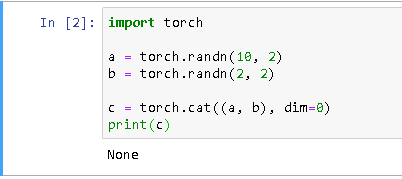# Torch.cat() returning None. (Solved by pytorch reinstallation)

I’ve been using torch.cat() for my unet implementation, but in pytorch 0.4.0 it has begun to return None and is defined as such:

def cat(param, dim):
return None

in torch’s init.py for me. How would I go about fixing this? I tried to use torch.stack() but it expects tensors to have the same shape. I’m getting this error:

RuntimeError: invalid argument 0: Sizes of tensors must match except in dimension 1. Got 512 and 256 in dimension 2 at c:\programdata\miniconda3\conda-bld\pytorch_1524549877902\work\aten\src\th\generic/THTensorMath.c:3586

from this line:

x = torch.stack((x, prev_feature_map), dim=1)

Hope to hear from you soon! And thank you in advance!

Could you tell me, where you have found the `init.py` defining `cat`?
Also, could you try to run the following code:

``````a = torch.randn(10, 2)
b = torch.randn(2, 2)

c = torch.cat((a, b), dim=0)
``````

and post the result?

1 Like

I’m getting this back: